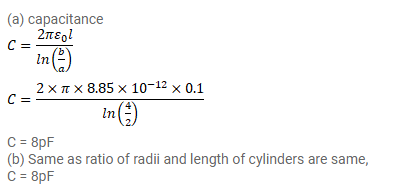# A cylindrical capacitor is constructed using two coaxial cylinders ofQuestion:

A cylindrical capacitor is constructed using two coaxial cylinders of the same length $10 \mathrm{~cm}$ and of radii $2 \mathrm{~mm}$ and $4 \mathrm{~mm}$.

(a) Calculate the Capacitance.

(b) Another capacitor of the same length is constructed with cylinders of radii $4 \mathrm{~mm}$ and $8 \mathrm{~mm}$. Calculate the capacitance.

Solution: